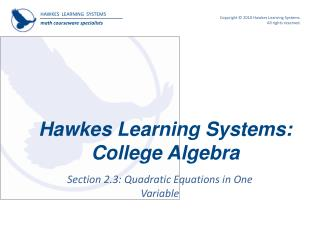DownloadDownload PresentationHawkes Learning Systems: College Algebra

# Hawkes Learning Systems: College Algebra

Download Presentation## Hawkes Learning Systems: College Algebra

- - - - - - - - - - - - - - - - - - - - - - - - - - - E N D - - - - - - - - - - - - - - - - - - - - - - - - - - -
##### Presentation Transcript

1. Hawkes Learning Systems:College Algebra Section 2.3: Quadratic Equations in One Variable

2. Objectives • Solving quadratic equations by factoring. • Solving “perfect square” quadratic equations. • Solving quadratic equations by completing the square. • The quadratic formula. • Interlude: gravity problems.

3. Quadratic Equations A quadratic equation in one variable, say the variable is an equation that can be transformed into the form Where , , and are real numbers and . Such equations are also called second-degree equations, as appears to the second power. The name quadratic comes from the Latin word quadrus, meaning “square”.

4. Solving Quadratic Equations by Factoring Let and represent algebraic expressions. If the product of and is , then at least one of and itself is . That is, For example, Zero –Factor Property

5. Solving Quadratic Equations by Factoring • The key to using factoring to solve a quadratic equation is to rewrite the equation so that appears by itself on one side of the equation. • If the trinomial can be factored, it can be written as a product of two linear factors and . • The Zero-Factor Property then implies that the only way for to be is if one (or both) of and is .

6. Example 1: Solving Quadratic Equations by Factoring Solve the quadratic equation by factoring. Step 1: Multiply both sides by . Step 2: Subtract from both sides so is on one side. Step 3: Factor and solve the two linear equations. or or

7. Example 2: Solving Quadratic Equations by Factoring Solve the quadratic equation by factoring. or In this example, the two linear factors are the same. In such cases, the single solution is called a double solution or a double root.

8. Example 3: Solving Quadratic Equations by Factoring Solve the quadratic equation by factoring.

9. Solving “Perfect Square” Quadratic Equations In some cases where the factoring method is unsuitable, the solution to a second-degree polynomial can be obtained by using our knowledge of square roots. Ifis an algebraic expression and if is a constant: If a given quadratic equation can be written in the form we can use the above observation to obtain two linear equations that can be easily solved. implies

10. Example 4: “Perfect Square” Quadratic Equations Solve the quadratic equation by taking square roots. Step 1: Take the square root of each side. Step 2: Subtract from both sides. Step 3: Divide both sides by .

11. Example 5: “Perfect Square” Quadratic Equations Solve the quadratic equation by taking square roots. In this example, taking square roots leads to two complex number solutions.

12. Solving Quadratic Equations by Completing the Square Method of Completing the Square Step 1: Write the equation in the form . Step 2: Divide byif, so that the coefficient of is : . Step 3: Divide the coefficient of by , square the result, and add this to both sides. Step 4: The trinomial on the left side will now be a perfect square. That is, it can be written as the square of an algebraic expression.

13. Example 6: Completing the Square Solve the quadratic equation by completing the square. Step 1: Move the constant term to the other side of the equation. Step 2: Divide by , the coefficient of . Step 3: Add to both sides. Step 4: Factor the trinomial, and solve.

14. Example 7: Completing the Square Solve the quadratic equation by completing the square.

15. The Quadratic Formula The method of completing the square can be used to derive the quadratic formula,a formula that gives the solution to any equation of the form . Step 1: Move the constant to the other side of the equation. Step 2: Divide by . Step 3: Divide by square the result, and add it to both sides of the equation.

16. The Quadratic Formula Deriving the quadratic formula (cont.). Step 4: Factor the trinomial, and solve for .

17. The Quadratic Formula The solutions of the equation are: Note: • The equation has real solutions if . • The equation has a double solution if . • The equation has complex solutions (which are conjugates of one another) if .